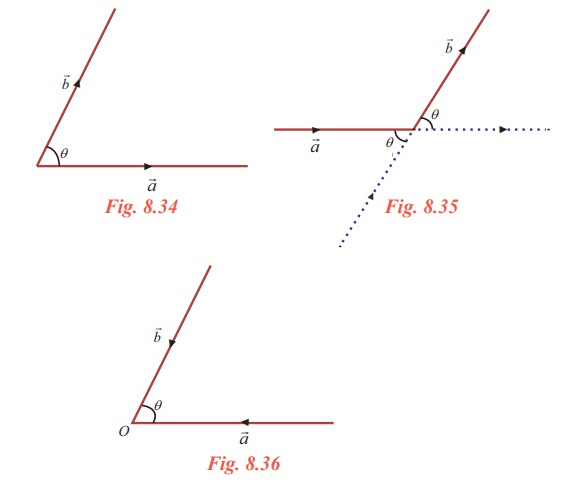Home | | Maths 11th std | Product of Vectors

# Product of Vectors

There are two ways of multiplying two vectors. (i) scalar product (dot product) and (ii) vector product (cross product).

Product of Vectors

We have seen the notion of addition of two vectors, subtraction of one vector from another vector and the multiplication of a vector by a scalar. Now we study the notion of product of two vectors. There are two ways of multiplying two vectors.

(i) scalar product (dot product) and

(ii) vector product (cross product).

To define such products we need the angle between two vectors.

Angle between two vectors

Letandbe any two vectors represented byandrespectively. Angle betweenandis the angle between their directions when these directions are either both converge as in Fig. 8.36 or both diverge as in Fig. 8.34 from their point of intersectionNote that, if q is the angle between two vectors then 0 ≤ θπ

When θ = 0 or π , the vectors are parallel.

If two vectors neither converge nor diverge as in Fig. 8.35 then we can make them into either converge or diverge by extending the length of the vectors to find the angle between the two vectors.

Tags : Mathematics , 11th Mathematics : UNIT 8 : Vector Algebra I
Study Material, Lecturing Notes, Assignment, Reference, Wiki description explanation, brief detail
11th Mathematics : UNIT 8 : Vector Algebra I : Product of Vectors | Mathematics